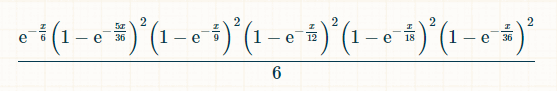# Bonus Craps

## Introduction

Bonus Craps is a set of three side bets found in craps. There are as follows:
• Small — Wins if the shooter rolls every total from 2 to 6, before rolling a total of 7. Wins usually pay 30 to 1.
• Tall — Wins if the shooter rolls every total from 8 to 12, before rolling a total of 7. Wins usually pay 30 to 1.
• All — Wins if the shooter rolls every total from 2 to 12, except a 7, before rolling a total of 7. Wins usually pay 150 to 1.

In 2021, I started to see these same bets called "Diceology" at the Venetian.

## Analysis

The following table shows my analysis of the Small and Tall bets, when wins pay 30 to 1. The lower right cell shows a house edge of 18.30%.

### Small and Tall

Event Pays Probability Return
Win 30 0.026354 0.790617
Loss -1 0.973646 -0.973646
Total   1.000000 -0.183029

In the past, some tables were known to pay 34 to 1 on the Small and Tall. These more generous odds have a house edge of 7.76%.

The following table shows my analysis of the All bet, when wins pay 150 to 1. The lower right cell shows a house edge of 20.61%.

### All

Event Pays Probability Return
Win 150 0.005258 0.788655
Loss -1 0.994742 -0.994742
Total   1.000000 -0.206087

In the past, some tables were known to pay 175 to 1 on the All. These more generous odds have a house edge of 7.47%.

## Methodology

I've analyzed Bonus Craps three ways, as follows:

• Simulation — This is probably the easiest way. However, for mathematical purists like me, simulations are always so intellectually unsatisfying.
• Markov Chain — This method is tedious and time consuming. For the Small and Tall it would require a 6x6 transition matrix and for the All a 12x12.
• Integral Calculus — This method is surprisginly easy with the use of an integral calculator. I explain it in more depth below.

Imagine that instead of significant events being determined by the roll of dice, one at a time, consider them as an instant in time. Assume the time between events has a memory-less property, with an average time between events of one unit of time. In other words, the time between events follows an exponetial distribution with a mean of 1. This will not matter for purposes of adjudicating the bet, because events still happen one at a time.

The following is the probability any given total has NOT been rolled at least once within x units of time:

• 2 or 12: exp(-x/36)
• 3 or 11: exp(-x/18)
• 4 or 10: exp(-x/12)
• 5 or 9: exp(-x/9)
• 6 or 8: exp(-5x/36)
• 7: exp(-x/6)

Let's look at the Small bet first. The odds are exactly the same for the Tall bet.

In x units of time, the probability that the 2, 3, 4, 5, and 6 have been rolled, and the 7 has not is: (1-exp(-x/36))*(1-exp(-x/18))*(1-exp(-x/12))*(1-exp(-x/9))*(1-exp(-5x/36))*exp(-x/6) The probability that at time x, the player rolls a 7, having previously rolled every total from 2 to 6, is:For the integration,I used this integral calculator.

Since a winning 7 could happen at any time, the probability of winning is the integral of this probability over x from 0 to infinity. Not that we need to know, but the integral is, before putting in the limits of integration is (-6*e^(-x/6)+(36*e^(-(7*x)/36))/7+(9*e^(-(2*x)/9))/2-(36*e^(-(11*x)/36))/11-3*e^(-x/3)-(36*e^(-(13*x)/36))/13+(18*e^(-(7*x)/18))/7+(12*e^(-(5*x)/12))/5+(9*e^(-(4*x)/9))/4-(36*e^(-(19*x)/36))/19-(9*e^(-(5*x)/9))/5+(12*e^(-(7*x)/12))/7)/6.

Putting in the limits of integration, the answer is 20049 / 760760 = apx. 0.02635390924864609.

Next, let's look at the All bet.

In x units of time, the probability that the 2, 3, 4, 5, 6, 8, 9, 10, 11, and 12 have been rolled, and the 7 has not is: (1-exp(-x/36))2*(1-exp(-x/16))2*(1-exp(-x/12))2*(1-exp(-x/9))2*(1-exp(-5x/36))2*exp(-x/6) The probability that at time x, the player rolls a 7, having previously rolled every total from 2 to 6, is:Since a winning 7 could happen at any time, the probability of winning is the integral of this probability over x from 0 to infinity. Not that we need to know, but the integral is, before putting in the limits of integration is (-6*e^(-x/6)+(72*e^(-(7*x)/36))/7+(9*e^(-(2*x)/9))/2-8*e^(-x/4)-(18*e^(-(5*x)/18))/5-(72*e^(-(11*x)/36))/11+(72*e^(-(13*x)/36))/13+(108*e^(-(7*x)/18))/7+(24*e^(-(5*x)/12))/5-(27*e^(-(4*x)/9))/4-(216*e^(-(17*x)/36))/17-10*e^(-x/2)-(72*e^(-(19*x)/36))/19+(27*e^(-(5*x)/9))/5+(144*e^(-(7*x)/12))/7+(54*e^(-(11*x)/18))/11-(72*e^(-(23*x)/36))/23-(15*e^(-(2*x)/3))/2-(216*e^(-(25*x)/36))/25-(54*e^(-(13*x)/18))/13+(8*e^(-(3*x)/4))/3+(54*e^(-(7*x)/9))/7+(72*e^(-(29*x)/36))/29-(72*e^(-(31*x)/36))/31-(9*e^(-(8*x)/9))/8-(24*e^(-(11*x)/12))/11+(18*e^(-(17*x)/18))/17+(72*e^(-(35*x)/36))/35-e^(-x))/6.

Putting in the limits of integration, the answer is 126538525259 / 24067258815600 = 0.0052577040961964420049.